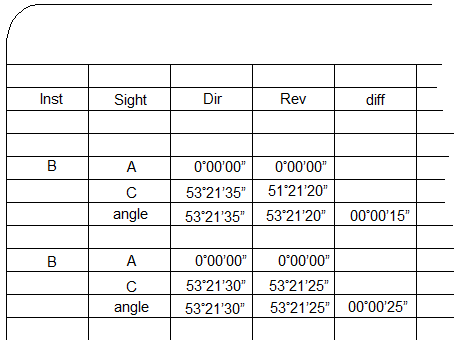## 3. Notes Clarity

Because it's easy to do on a TSI, it's tempting to zero the horizontal angle reading on each BS sighting, Figure C-4.Figure C-4 Resetting Angle

While easier to calculate the angle, it can be confusing to a third party. How were the measurements made?

As two complete D/R sets, or,

Two direct measurements first rotating back and forth between A and C, then two reverse measurements rotating back and forth between A and C?

Allowing the angle to accumulate without zeroing intermediate BS sightings makes it clear the order in which the measurements are made.

### b. Mistake

Inevitably, a mistake is made, either reading the instrument, sighting, or incorrectly locking/unlocking the angle display. This will show in the notes as an angle with an excessive D/R difference. Figure C-5 shows a mistaken reading and how to correctly deal with it.The large difference for the second D/R set is an indication of a mistake. Upon closer investigation, it looks like the mistake occurred on the second direct angle as the second reverse angle "fits" with the first D/R set.The initial inclination is to toss the second direct angle and keep the second reverse angle.However, accepting the reverse angle without a corresponding direct one doesn't compensate instrumental errors. The correct way to address the mistake is to reject the entire second D/R set..... and measure a third D/R set. Figure C-5 Correcting An Angle Measurement Error